Name:    1.G.1

Multiple Choice
Identify the choice that best completes the statement or answers the question.

1.

How many trapezoids were used in this picture?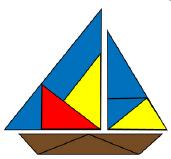a. 4 c. 0 b. 2 d. 3

2.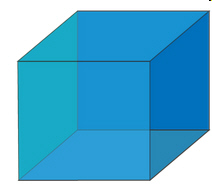How many rectangles are used in this picture?
 a. 8 c. 4 b. 6 d. 9

3.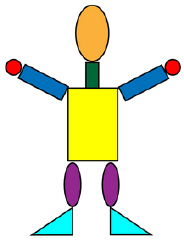How many rectangles are there in this picture?
 a. 1 c. 4 b. 3 d. 2

4.

How many circles in this picture?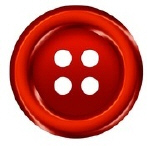a. 2 c. 5 b. 4 d. 6

5.

How many circles in this picture?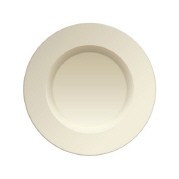a. 2 c. 3 b. 1 d. 0

6.

Which of the following shapes can be made by putting two triangles together?
 a. trapezoid c. oval b. circle d. rhombus

7.

Which sign is a rhombus?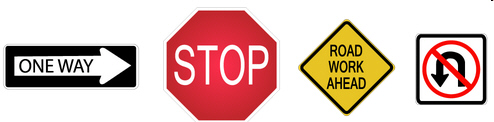a.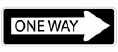c.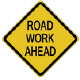b.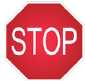d.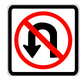8.

How many signs are rhombuses?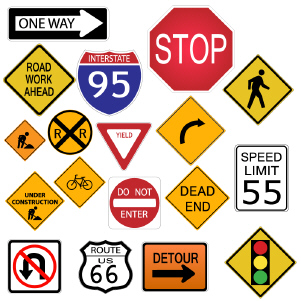a. 2 c. 6 b. 8 d. 4

9.

How many triangles were used in this picture?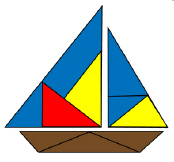a. 8 c. 13 b. 11 d. 9

10.

How many triangles were used in this picture?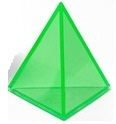a. 3 c. 4 b. 2 d. 5